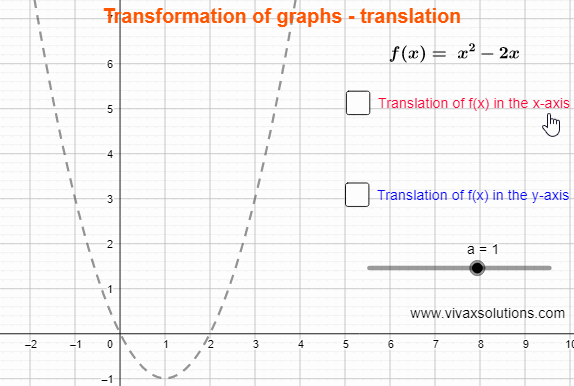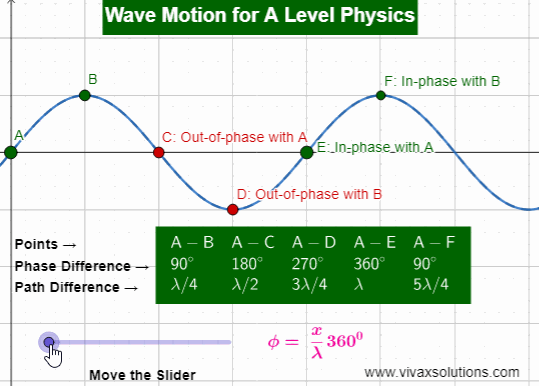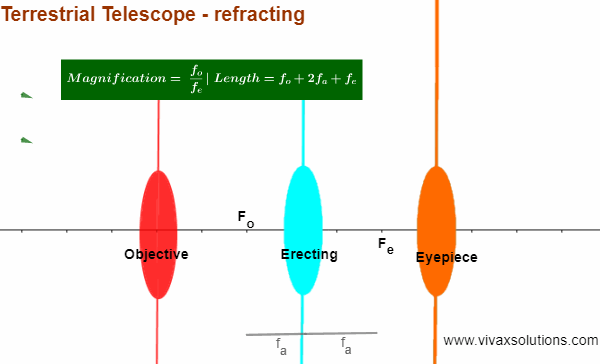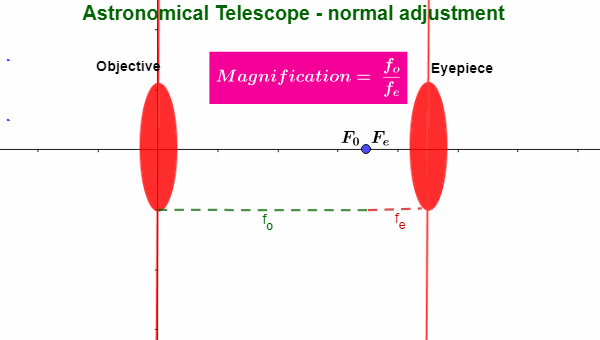## Posts

Showing posts from January 30, 2022

### Transformation of Graphs - translationf(x) → f(x + a) - a translation in the negative x-axis by a f(x) → f(x - a) - a translation in the positive x-axis by a   f(x) → f(x) +  a - a translation in the positive y-axis by a f(x) → f(x) -  a - a translation in the negative y-axis by a E.g.1 Sketch y = x 2 + 2x - 3 and find the following: The line of symmetry The solutions when y = 0 The coordinates of the minimum point The y-intercept   y = x 2 + 2x - 3 By completing the square, y = (x + 1) 2 - 4 1) Line of symmetry, x = -1 2) (x + 1) 2 - 4 = 0 → x = -3 or x = 1 3) Turning point ~ (-1,-4) 4) y-intercept ~ (0,-3) E.g.2 Sketch y = x 2  -4x + 3 and find the following: The line of symmetry The solutions when y = 0 The coordinates of the minimum point The y-intercept   y = x 2  -4x + 3 By completing the square, y = (x - 2) 2  - 1 1) Line of symmetry, x = 1 2) (x - 2) 2  - 1 = 0 → x = 3 or x = 1 3) Turning point ~ (2,-1) 4) y-intercept ~ (0,3) You can practise translation interactively with the following applet:

### Phase Difference between Two Points on a Wave and Path Difference Explained - interactiveIn the above animation, five points on the wave are considered for the explanation.  The fully interactive applet is given below for you to practise. The phase of a point implies its direction of vibration on a wave. For example, both points A and E vibrate exactly the same way are said to be in phase: when A goes up so does B; the phase difference is either 0 0 or 360 0 . If you consider the motion of points A and C, on the other hand, when A goes down C goes up or vice versa. Therefore, A and C are said to be out of phase; the phase difference is 180 0 . That means the phase difference between two points on a wave - or two waves for that matter - can take any value between 0 0 and 360 0 .  The phase difference between points A and B, for instance, is 90 0 . From the above examples, it is clear there is a connection between the path difference between two points - or two waves - and phase difference. It is as follows: Phase difference = (path difference / wavelength) x 360 φ = x

### Terrestrial Telescope - refracting telescope explained with interactive animationThe above animation is a terrestrial telescope, a refracting telescope. It has evolved from another refracting telescope, the astronomical telescope that produces an inverted image, making it not suitable for viewing objects on the Earth. The terrestrial telescope has addressed the issue to some extent: it has a third lens in auxiliary capacity, just to erect the image formed by the objective lens. When the second image is formed at the focus of the eyepiece, the final image is formed at infinity for viewing, while minimizing the strain on the eyes. Length of the telescope = f o + 2f a + f e The length of the telescope, however, can be minimized by letting the image from the objective formed at a distance of 2 x focal length of the erecting lens, that in turn forms the image at the same distance on the opposite side of the lens. The magnification, however, remains that of the astronomical telescope, when in the normal adjustment. Magnification = f o /f e Understandably, if f o is

### Astronomical Telescope Explained - with interactive practiceAn astronomical telescope is a refractiong telescope that consists of two convex lenses - the objective lens and the eyepiece. The objective lens gathers light from a distant object and the image formed, is viewed through the eyepiece. When the final image is formed in infinity, the rays enter the eyes through the eyepiece in parallel with each other, which in turn minimize the strain on the eyes; the telescope is said to be in the normal adjustment .  When the telescope is in the normal adjustment, the distance between the two lenses - or the length of the telescope -  is the sum of the focal lenghts.  The magnification is eqaul the ratio of the two focal lengths. By moving the eyepiece slightly towards the objective lens, the final image can be made to appear at a distance of 25 cm from the eye too. This, however, can lead to straining eyes. One of the setbacks of the astronomical telescope is the fact that the final image is inverted. Therefore, it is not good for viewing terrest